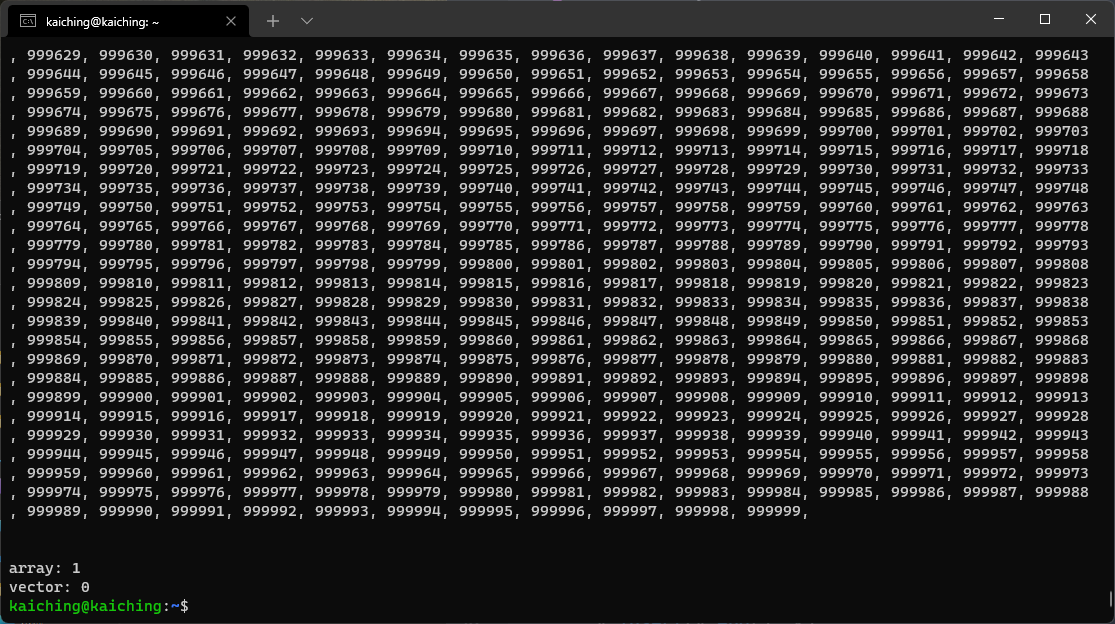# 單元 23 - 認識標準程式庫`cout << endl << m << endl << endl << endl;`

`int a = rand() % 10;`

```// 初始化密碼表字串
code_array = "abcdefghijklmnopqrstuvwxyz";
// 獲取時鐘週期個數
unsigned int seed = system_clock::now().time_since_epoch().count();
// 攪亂字串中的元素順序
shuffle(code_array.begin(), code_array.end(), mt19937(seed));```

`r += code_array.at(alphabet_array.find(c));`

14陣列、資料型態
15陣列、型態轉換
16vector 、擬隨機數
17vector 、 shuffle
18map 、時間相關
19map 、格式化輸出
20set 、數學相關
21集合體型態、數學相關
22命令列參數、系統指令
23輸入與檔案處理
24字串相關

```// 引入標準程式庫中時間日期的相關程式
#include <ctime>
// 引入標準程式庫中相關的輸入、輸出程式
#include <iostream>
// 引入標準程式庫中的 array
#include <array>
// 引入標準程式庫中的 vector
#include <vector>

// cout 為 std 中的輸出物件
using std::cout;
// endl 為 std 中的斷行符號
using std::endl;
// array 為 std 中的陣列型態
using std::array;
// vector 為 std 中的集合體型態
using std::vector;

int main() {
// 計算陣列處理時間
time_t t1 = time(NULL);
long i = 0;
array<long, 1000000> a;
for (int j: a) {
j = i++;
cout << j << ", ";
}
cout << endl;

time_t t2 = time(NULL);

// 計算 vector 處理時間
time_t t3 = time(NULL);
vector<long> v;
for (int k = 0; k < 1000000; k++) {
v.insert(v.end(), k);
cout << v[k] << ", ";
}
cout << endl;

time_t t4 = time(NULL);

// 顯示處理時間
cout << endl << endl;
cout << "array: " << t2 - t1 << endl;
cout << "vector: " << t4 - t2 << endl;

return 0;
}

/* 《程式語言教學誌》的範例程式
http://kaiching.org/
檔名：time_test.cxx
功能：示範利用標準程式庫比較 array 與 vector 執行時間
作者：張凱慶 */```

```// 顯示處理時間
cout << endl << endl;
cout << "array: " << t2 - t1 << endl;
cout << "vector: " << t4 - t2 << endl;```

`time_t t1 = time(NULL);`1. 標準程式庫是依 C++ 標準隨編譯器提供的程式庫，第三方程式庫則是其他開發商提供程式庫。
2. 標準程式庫提供眾多的應用，包括容器、工具、地方資訊、字串、串流及輸出入、語言、執行緒、數學等應用。
3. 學習程式設計不應該重新發明輪子，實際寫程式往往會混用程式庫的內容。

1. 為什麼要有標準程式庫？不能程式的所有功能都自己開發嗎？
2. 什麼是命名空間？為什麼要用命名空間？
3. 為什麼要有第三方程式庫？除了 Qt 外還有其他的第三方程式庫嗎？

1. 標準程式庫 iostreamcin 物件用來接收使用者輸入，連帶須使用 >> 運算子，將儲存輸入的變數放在 >> 之後，寫一個程式 exercise2301.cxx ，宣告字元變數 c ，然後用 cin 接收使用者輸入，最後印出字元變數 c參考程式碼
2. 承上題，寫一個程式 exercise2302.cxx ，改成使用 cin 接收使用者輸入兩個整數，然後印出相加值。 參考程式碼
3. 承上題，寫一個程式 exercise2303.cxx ，利用 while (true) 迴圈接收使用者輸入，直到使用者輸入 'q' 之後就結束程式。 參考程式碼
4. 標準程式庫中的 fstream 可以用來進行檔案處理，寫一個程式 exercise2304.cxx ，宣告 fstream 的檔案物件，然後利用 open() 開啟指定的檔案， open() 的第一個參數為檔名字串，第二個參數可以用 std::ios_base::app ，接下來用 is_open() 判斷檔案是否開啟，開啟成功寫入自訂字串，開啟失敗印出失敗訊息。 參考程式碼